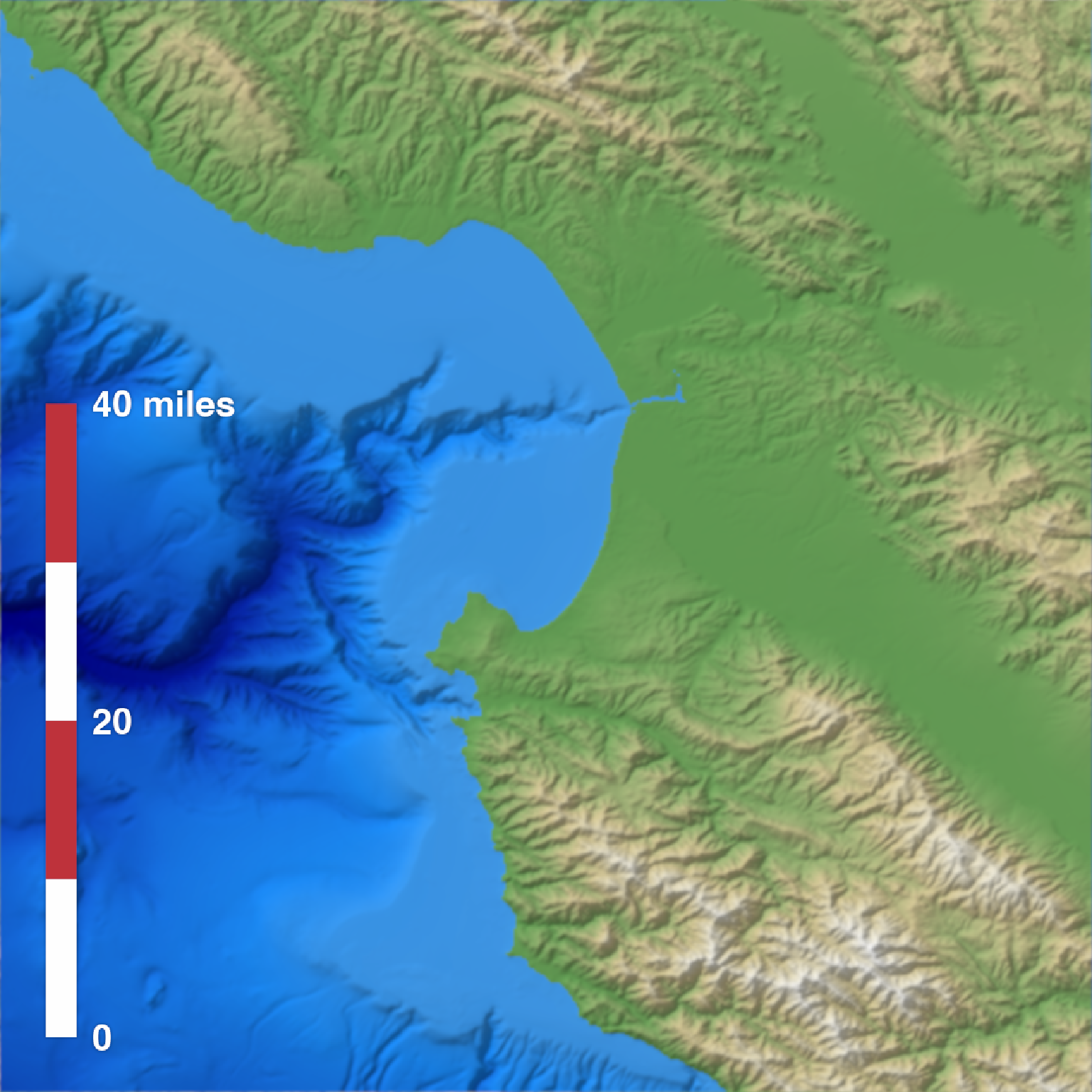This function creates an overlay with a scale bar of a user-specified length. It uses the coordinates of the map (specified by passing an extent) and then creates a scale bar at a specified x/y proportion across the map. If the map is not projected (i.e. is in lat/long coordinates) this function will use the geosphere package to create a scale bar of the proper length.

generate_scalebar_overlay(
extent,
length,
x = 0.05,
y = 0.05,
latlong = FALSE,
thickness = NA,
bearing = 90,
unit = "m",
flip_ticks = FALSE,
labels = NA,
text_size = 1,
decimals = 0,
text_offset = 1,
heightmap = NULL,
width = NA,
height = NA,
color1 = "white",
color2 = "black",
text_color = "black",
font = 1,
border_color = "black",
tick_color = "black",
border_width = 1,
tick_width = 1,
halo_color = NA,
halo_expand = 1,
halo_alpha = 1,
halo_offset = c(0, 0),
halo_blur = 1
)

## Arguments

extent

Either an object representing the spatial extent of the scene (either from the raster, terra, sf, or sp packages), a length-4 numeric vector specifying c("xmin", "xmax","ymin","ymax"), or the spatial object (from the previously aforementioned packages) which will be automatically converted to an extent object. If this is in lat/long coordinates, be sure to set latlong = TRUE.

length

The length of the scale bar, in units. This should match the units used on the map, unless extent uses lat/long coordinates. In that case, the distance should be in meters.

x

Default 0.05. The x-coordinate of the bottom-left corner of the scale bar, as a proportion of the full map width.

y

Default 0.05. The y-coordinate of the bottom-left corner of the scale bar, as a proportion of the full map height.

latlong

Default FALSE. Set to TRUE if the map is in lat/long coordinates to get an accurate scale bar (using distance calculated with the geosphere package).

thickness

Default NA, automatically computed as 1/20th the length of the scale bar. Width of the scale bar.

bearing

Default 90, horizontal. Direction (measured from north) of the scale bar.

unit

Default m. Displayed unit on the scale bar.

flip_ticks

Default FALSE. Whether to flip the ticks to the other side of the scale bar.

labels

Default NA. Manually specify the three labels with a length-3 character vector. Use this if you want display units other than meters.

text_size

Default 1. Text size.

decimals

Default 0. Number of decimal places for scale bar labels.

text_offset

Default 1. Amount of offset to apply to the text from the scale bar, as a multiple of thickness.

Default 0.5, centered. Text justification. 0 is left-justified, and 1 is right-justified.

heightmap

Default NULL. The original height map. Pass this in to extract the dimensions of the resulting RGB image array automatically.

width

Default NA. Width of the resulting image array. Default the same dimensions as height map.

height

Default NA. Width of the resulting image array. Default the same dimensions as height map.

color1

Default black. Primary color of the scale bar.

color2

Default white. Secondary color of the scale bar.

text_color

Default black. Text color.

font

Default 1. An integer which specifies which font to use for text. If possible, device drivers arrange so that 1 corresponds to plain text (the default), 2 to bold face, 3 to italic and 4 to bold italic.

border_color

Default black. Border color of the scale bar.

tick_color

Default black. Tick color of the scale bar.

border_width

Default 1. Width of the scale bar border.

tick_width

Default 1. Width of the tick.

halo_color

Default NA, no halo. If a color is specified, the text label will be surrounded by a halo of this color.

halo_expand

Default 1. Number of pixels to expand the halo.

halo_alpha

Default 1. Transparency of the halo.

halo_offset

Default c(0,0). Horizontal and vertical offset to apply to the halo, as a proportion of the full scene.

halo_blur

Default 1. Amount of blur to apply to the halo. Values greater than 30 won't result in further blurring.

## Value

Semi-transparent overlay with a scale bar.

## Examples

if(run_documentation()) {
#Create the water palette
water_palette = colorRampPalette(c("darkblue", "dodgerblue", "lightblue"))(200)
bathy_hs = height_shade(montereybay, texture = water_palette)
#Set scalebar font
par(family = "Arial")

#Generate flat water heightmap
mbay = montereybay
mbay[mbay < 0] = 0

base_map = mbay %>%

#For convenience, the extent of the montereybay dataset is included as an attribute
mb_extent = attr(montereybay, "extent")

base_map %>%
add_overlay(generate_scalebar_overlay(extent = mb_extent, length = 40000,
heightmap = montereybay,
latlong=TRUE)) %>%
plot_map()
}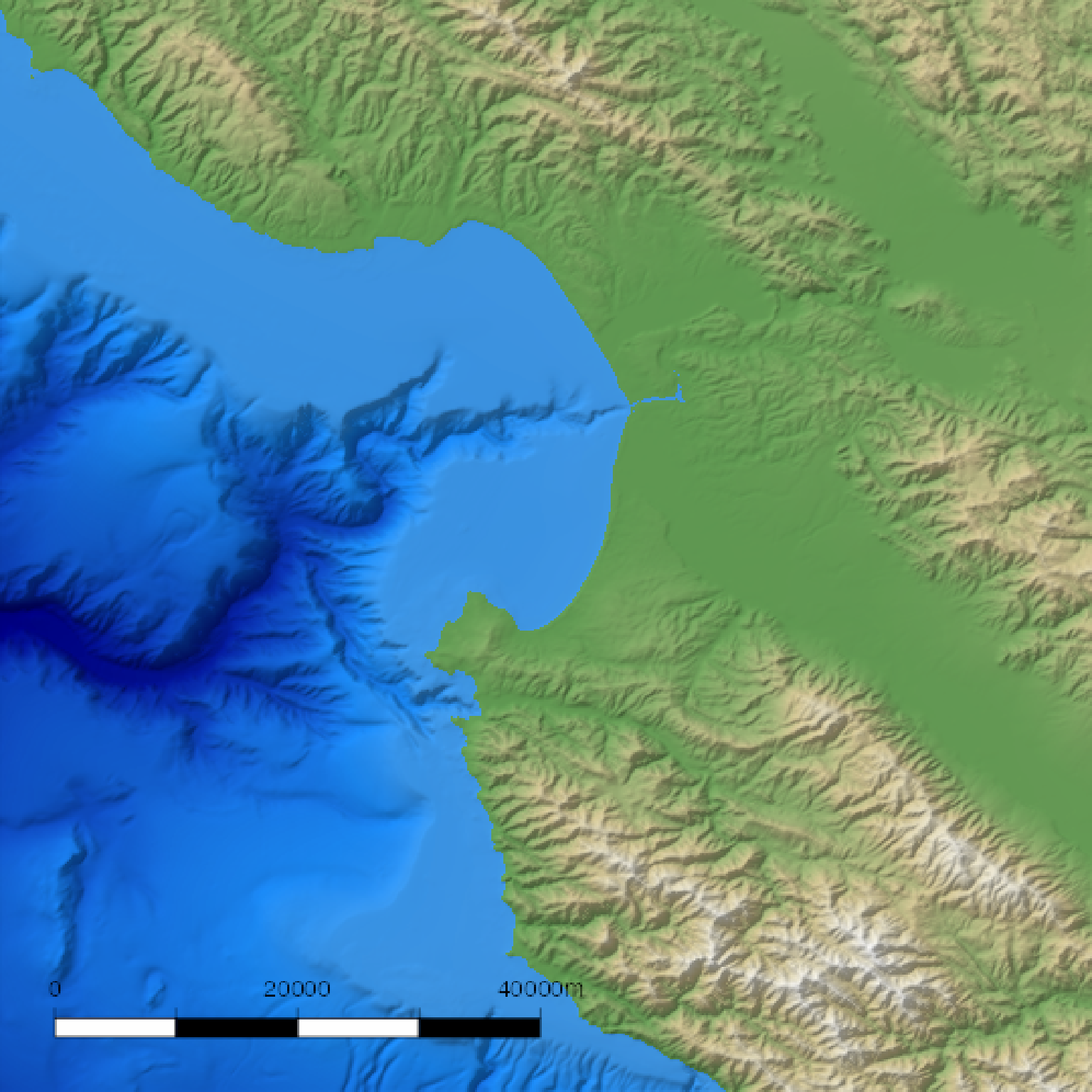if(run_documentation()) {
#Change the text color
base_map %>%
add_overlay(generate_scalebar_overlay(extent = mb_extent, length = 40000,
text_color = "white",
heightmap = montereybay,
latlong=TRUE)) %>%
plot_map()
}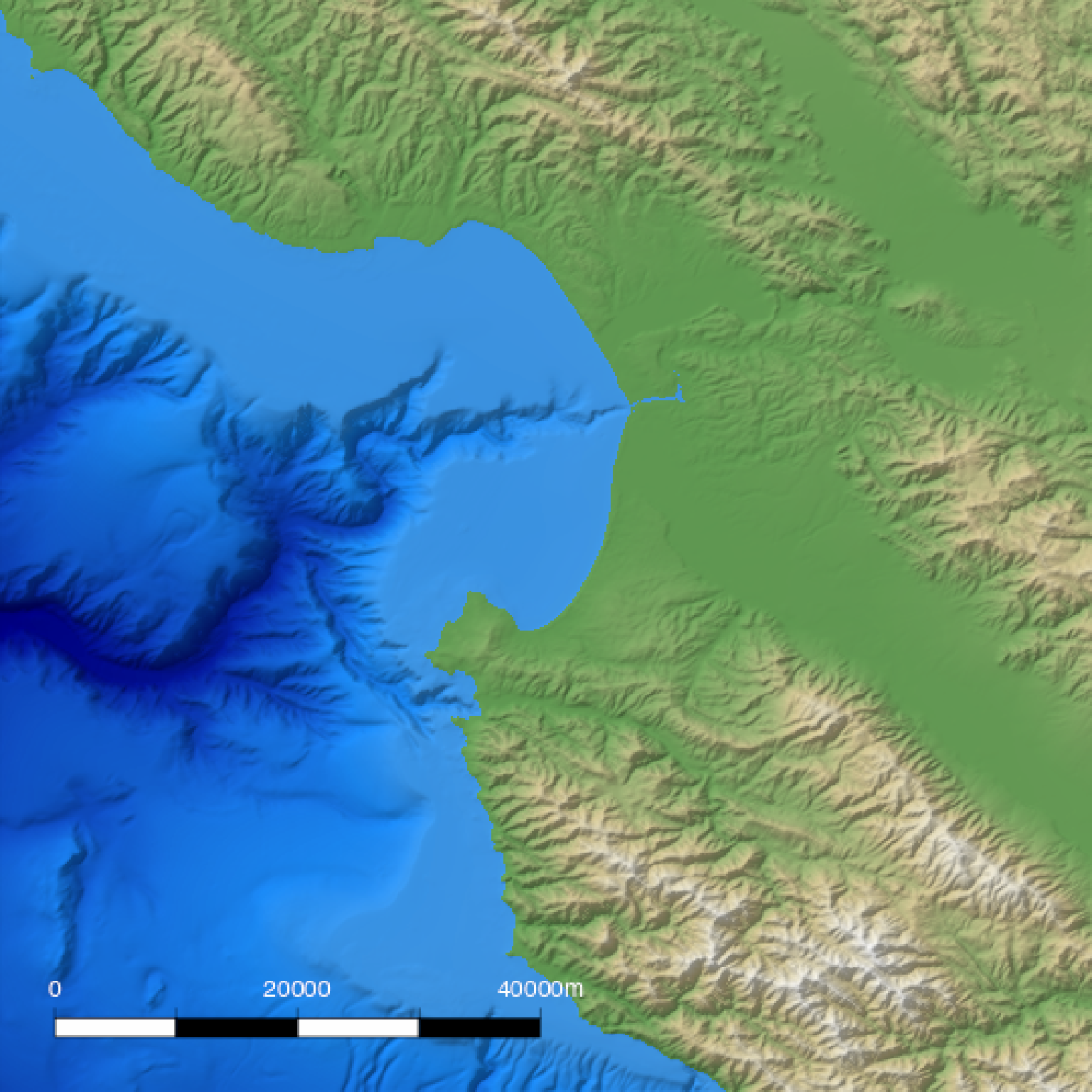if(run_documentation()) {
#Change the length
base_map %>%
add_overlay(generate_scalebar_overlay(extent = mb_extent, length = 30000,
text_color = "white",
heightmap = montereybay,
latlong=TRUE)) %>%
plot_map()
}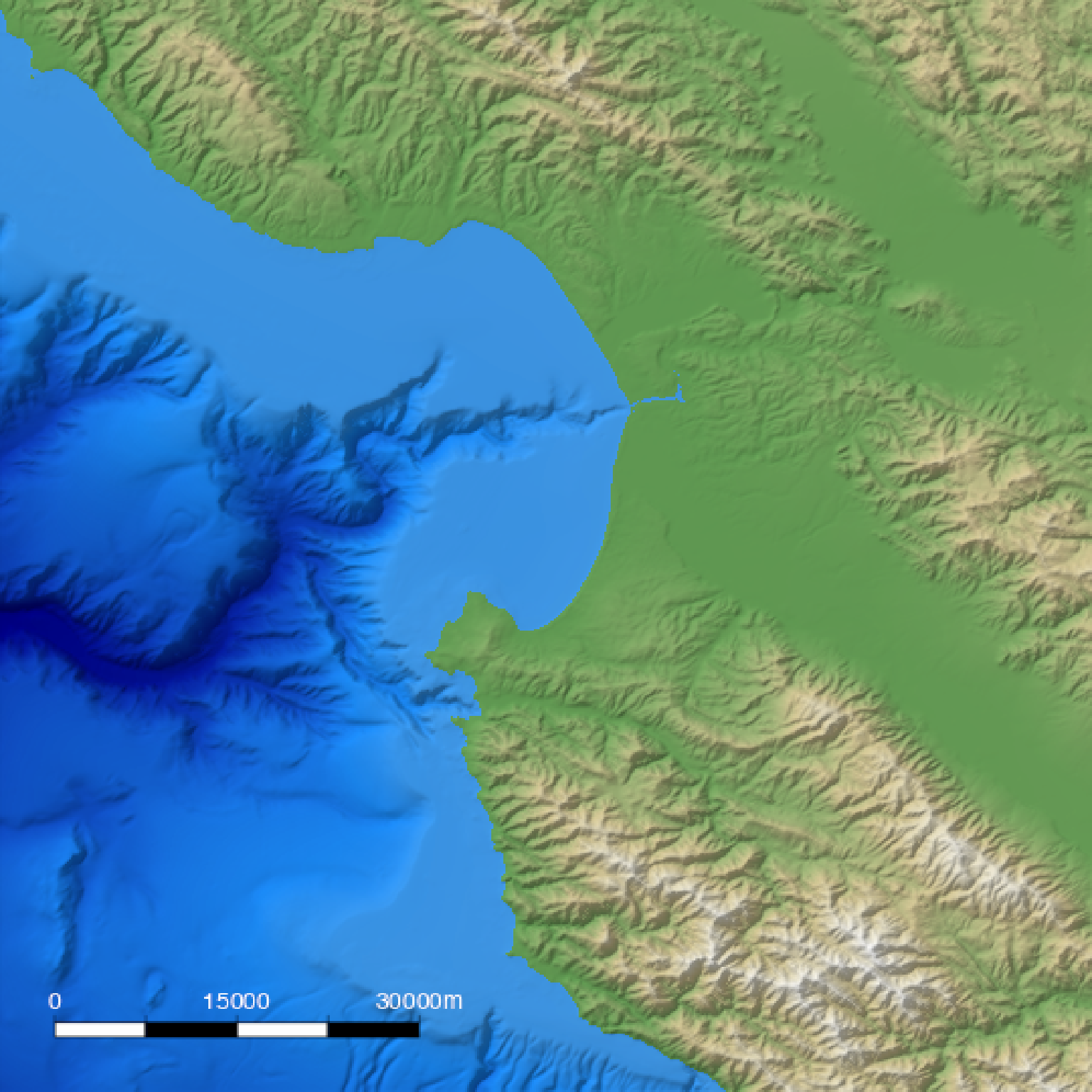if(run_documentation()) {
#Change the thickness (default is length/20)
base_map %>%
add_overlay(generate_scalebar_overlay(extent = mb_extent, length = 30000,
text_color = "white", thickness = 30000/10,
heightmap = montereybay,
latlong=TRUE)) %>%
plot_map()
}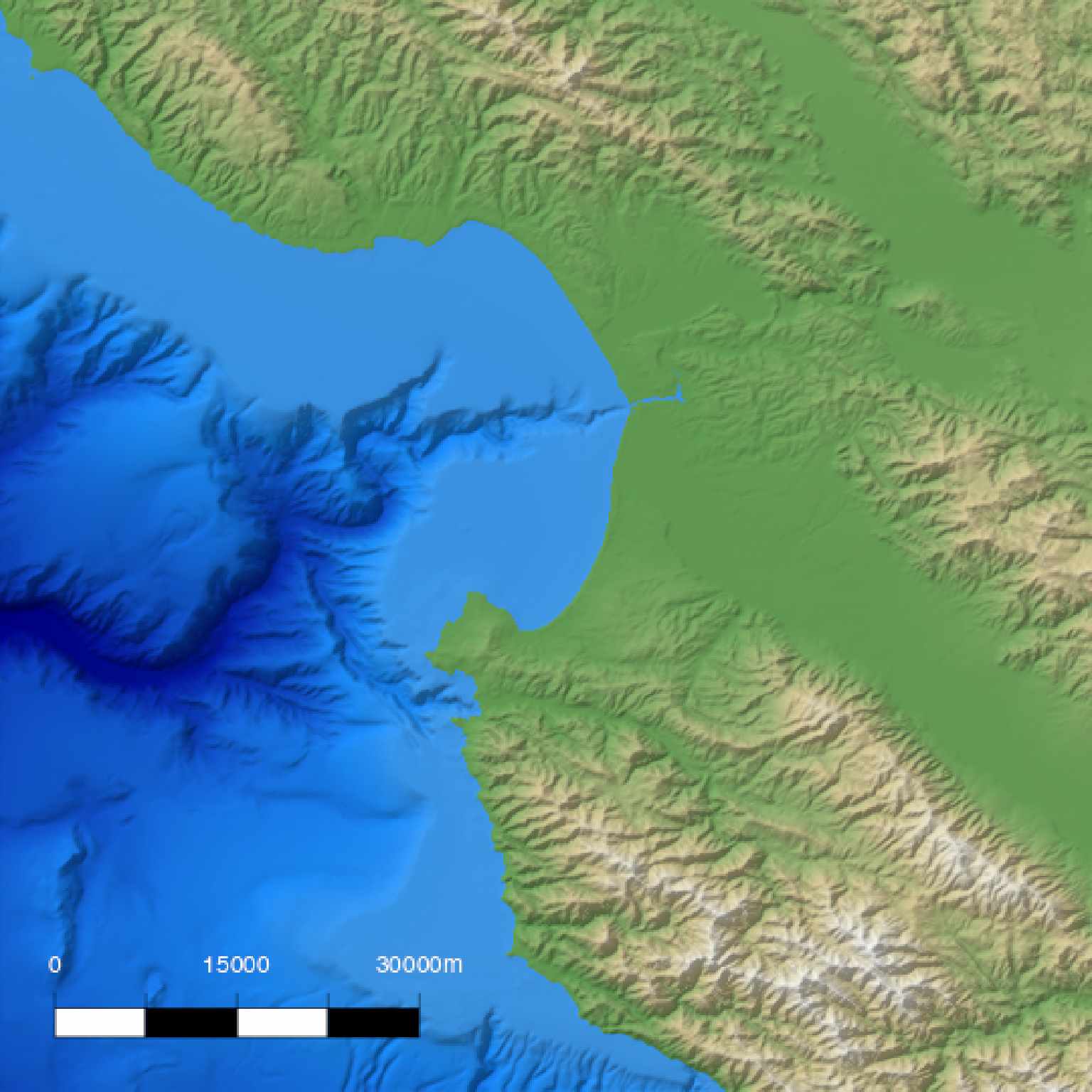if(run_documentation()) {
#Change the text offset (given in multiples of thickness)
base_map %>%
add_overlay(generate_scalebar_overlay(extent = mb_extent, length = 30000,
text_color = "white", thickness = 30000/10,
text_offset = 0.75,
heightmap = montereybay,
latlong=TRUE)) %>%
plot_map()
}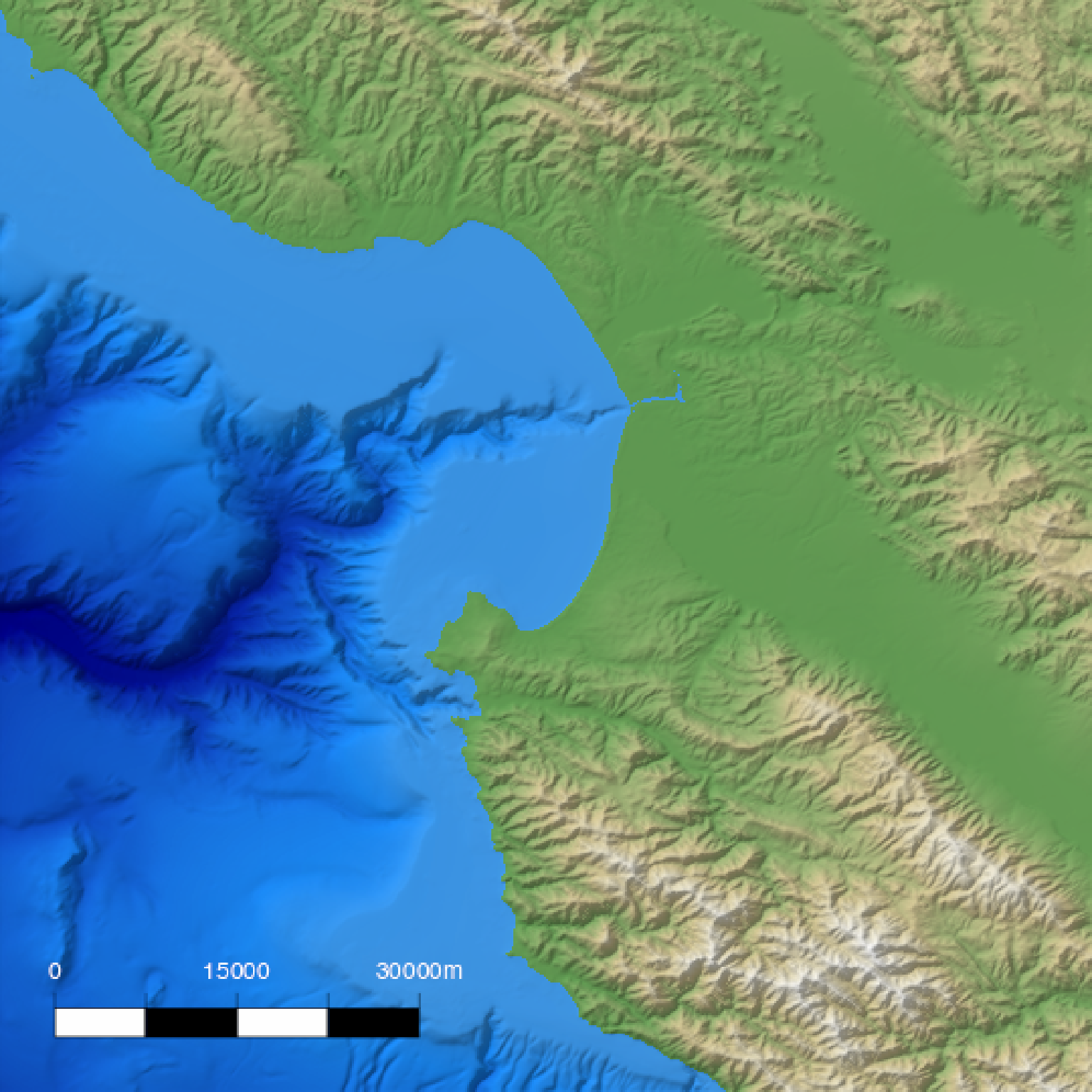if(run_documentation()) {
#Change the primary and secondary colors, along with the border and tick color
base_map %>%
add_overlay(generate_scalebar_overlay(extent = mb_extent, length = 30000,
text_color = "white", border_color = "white",
tick_color = "white",
color1 = "darkolivegreen4", color2 = "burlywood3",
heightmap = montereybay,
latlong=TRUE)) %>%
plot_map()
}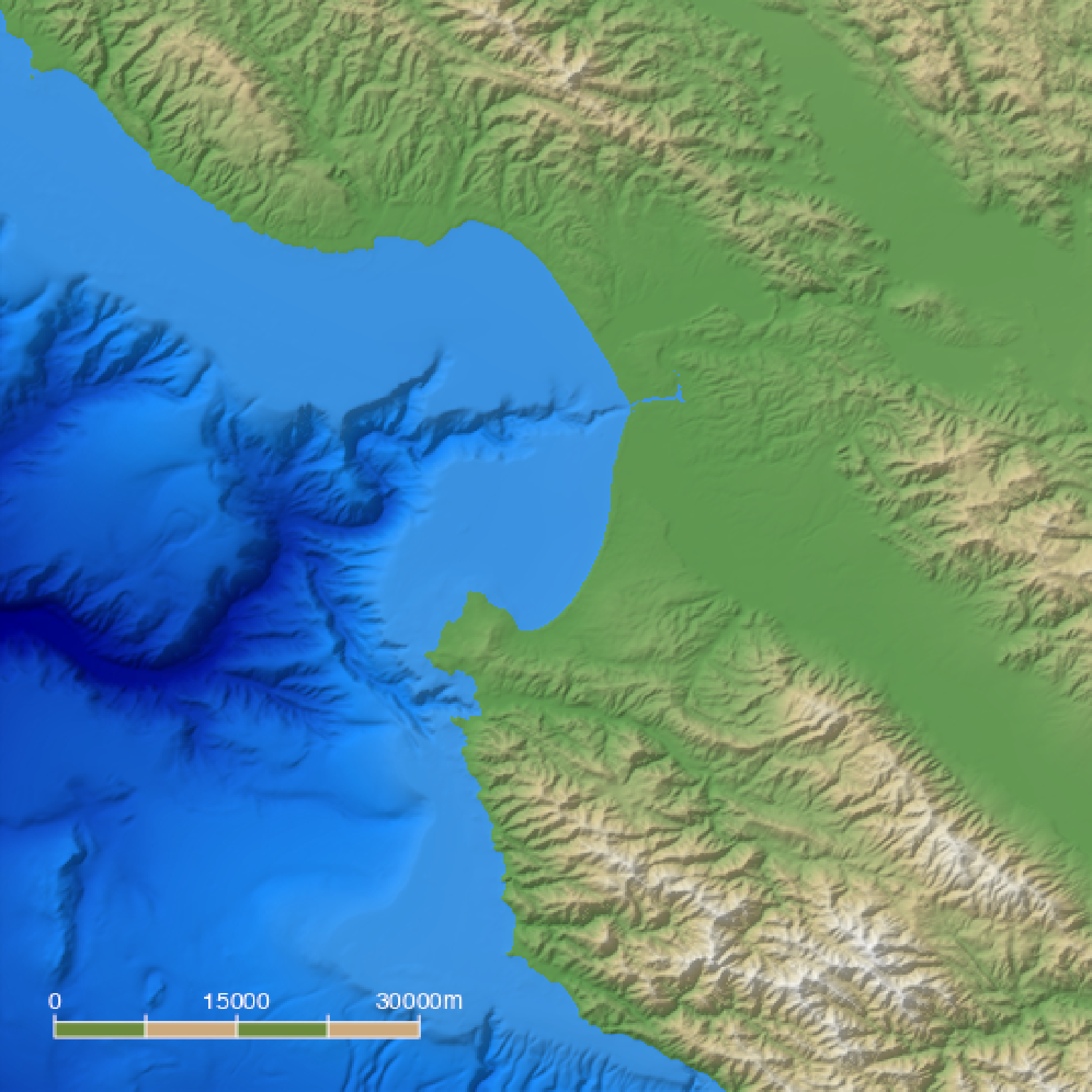if(run_documentation()) {
base_map %>%
add_overlay(generate_scalebar_overlay(extent = mb_extent, length = 40000,
halo_color = "white", halo_expand = 1,
heightmap = montereybay,
latlong=TRUE)) %>%
plot_map()
}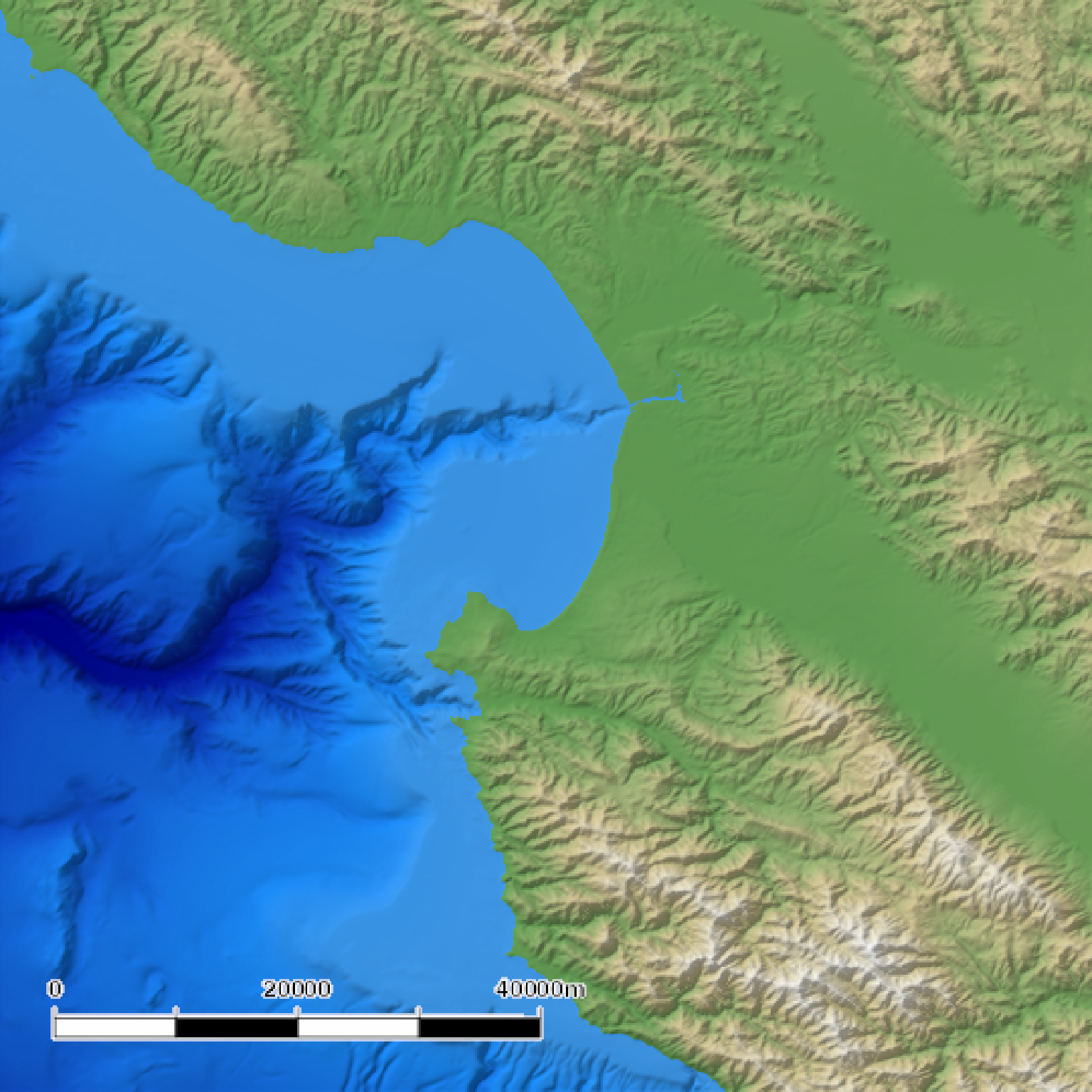if(run_documentation()) {
#Change the orientation, position, text alignment, and flip the ticks to the other side
base_map %>%
add_overlay(generate_scalebar_overlay(extent = mb_extent, length = 40000, x = 0.07,
bearing=0, adj = 0, flip_ticks = TRUE,
halo_color = "white", halo_expand = 1.5,
heightmap = montereybay,
latlong=TRUE)) %>%
plot_map()
}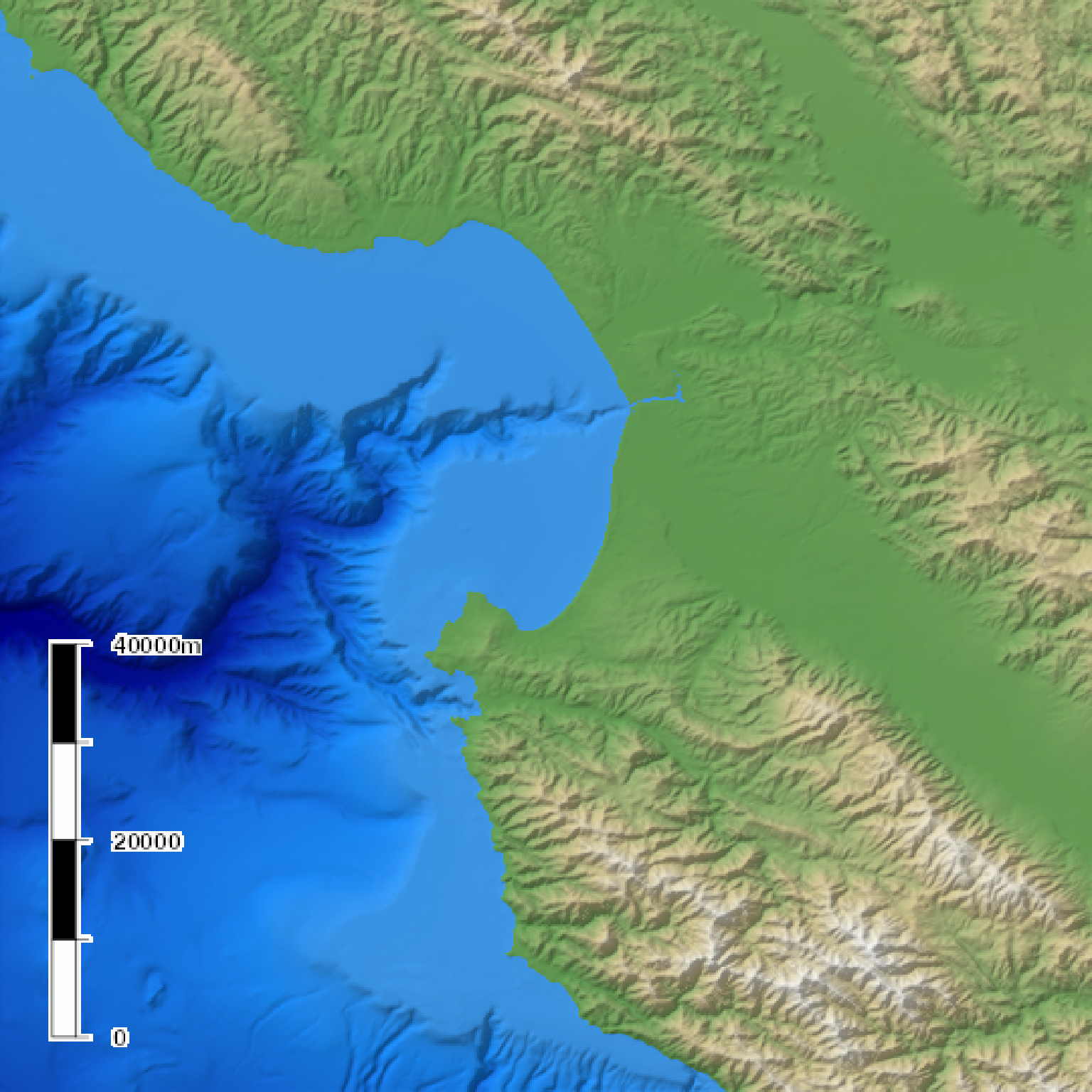if(run_documentation()) {
#64373.8 meters in 40 miles
#Create custom labels, change font and text size, remove the border/ticks, and change the color
#Here, we specify a width and height to double the resolution of the image (for sharper text)
base_map %>%
add_overlay(generate_scalebar_overlay(extent = mb_extent, length = 64373.8, x = 0.07,
labels = c("0", "20", "40 miles"), thickness=2500,
text_size=3, font = 2, text_offset = 0,
text_color="white", color2="#bf323b", border_color=NA,
tick_color="red", tick_width=0,
bearing=0, adj = 0, flip_ticks = TRUE,
halo_color="black", halo_blur=3, halo_alpha=0.5,
width = ncol(montereybay)*2,
height = nrow(montereybay)*2,
latlong=TRUE), rescale_original=TRUE) %>%
plot_map()
}